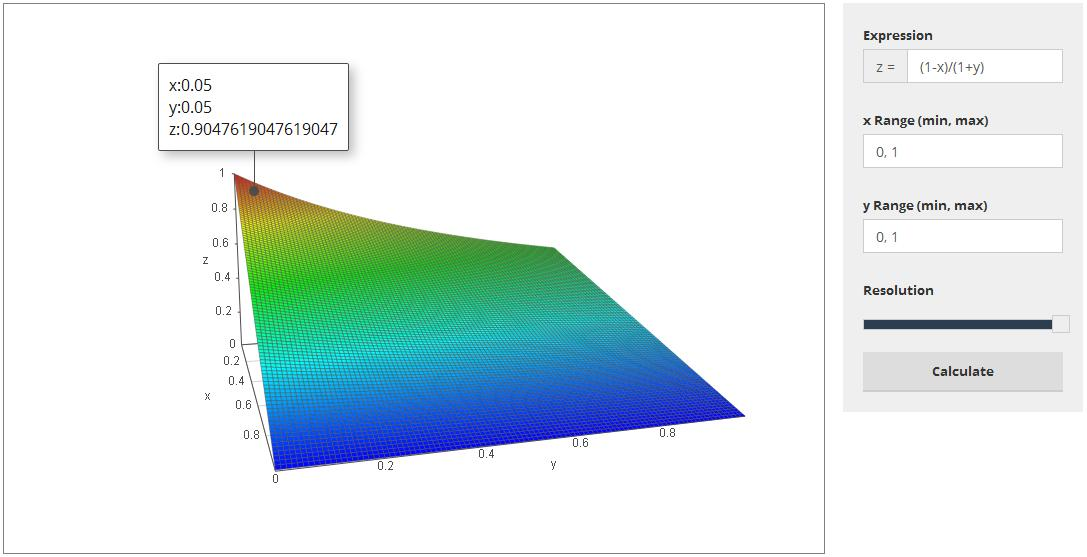If you find any of this useful, please consider donating via PayPal to help keep this site going.# A More Realistic Gold to Silver Ratio

1/25/17

Many gold and silver investors like to talk about the gold to silver ratio, or G/S. The interpretation is, if you sell 1oz gold, how many oz of silver can you buy.

What do I mean? Consider the current G/S = 71.04. If I sell 1oz gold for the current spot of \$1,197.70, I can buy 71.04 oz of silver at the current spot of \$16.86.

Wrong. This G/S ratio is very naive, because it ignores real-life frictions of buying and selling (not to mention other costs such as shipping and storage, as well as foreign exchange rates, but I won't even go there). Companies will charge you a certain percent to sell your gold, and a certain percent to sell you silver. They do this because they are a company (profit seeking) and they are market makers. In other words, they need to pay bills and have a little leftover as well as if they didn't exist you couldn't sell your precious metals (or at least not sell them as easily or as cheaply).

Let's assume that the percent you get charged to sell your 1oz gold is X and the percent you get charged to buy silver is Y. What will happen is, G/S will be reduced to [G(1-X)]/[S(1+Y)] or (G/S)*[(1-X)/(1+Y)]. The interpretation of the ratio (1-X)/(1+Y) is, if you thought you'd get G/S silver ounces at the end of your transactions, you are only getting (1-X)/(1+Y) percent of that. Put another way, you are getting 1-[(1-X)/(1+Y)] percent less.

Here is a graph of the function z = (1-X)/(1+Y). We can see that for small-ish X and Y, (1-X)/(1+Y) isn't too bad. However, as X and Y increase, (1-X)/(1+Y) decreases considerably.Ok, let's see this in action with some actual numbers. Let's assume the current gold and silver spot as mentioned above, and X = 5% and Y = 5%. What you will be able to sell your 1oz of gold for goes from \$1,197.70 to \$1,197.70*(1-.05) = \$1,197.70*.95 = \$1,137.82. This means you just lost \$59.89.

For silver, what you are being charged to buy went from \$16.86 to \$16.86*(1+.05) = \$16.86*1.05 = \$17.70 per oz. In other words, you have just been charged \$.84 extra per silver oz.

If you now calculate G/S, you get G/S = \$1,137.82/\$17.70 = 64.27.

We see two things here. First, this represents a \$.84 * 64.27 = \$54.18 total loss in silver. Second, this is 71.04 - 64.27 = 6.77 oz silver less than you thought you were getting using the naive G/S. Your total loss in dollars is \$59.89 + \$54.18 = \$114.07.

Note that the percents I used were both 5%. In real life these percents are often different. Second, while these percents can be less than 5%, they can also be higher than 5%. For example, if I use X = Y = 10%, we see that (1-X)/(1+Y) = 81.82%, and the new G/S is 58.12.

These percentages X and Y will definitely fluctuate, so be sure to calculate (G/S)*[(1-X)/(1+Y)] instead of just the naive G/S. If there is some spike in X or Y...watch out if you only think in terms of the naive G/S.

The way, of course, around this, is to only sell your gold if the gold spot and X work for you. Also, wait before buying silver. Nothing says that you have to immediately buy silver after selling your gold. Simply wait until the silver spot and Y work for you.